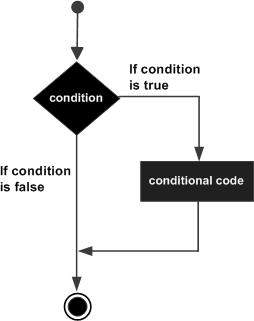# R - If Statement

#### R Programming Online Training

40 Lectures 1.5 hours

#### Support Vector Machines in R (SVM in R)

27 Lectures 3 hours

#### Data Visualization using R Programming

14 Lectures 10 hours

An if statement consists of a Boolean expression followed by one or more statements.

## Syntax

The basic syntax for creating an if statement in R is −

```if(boolean_expression) {
// statement(s) will execute if the boolean expression is true.
}
```

If the Boolean expression evaluates to be true, then the block of code inside the if statement will be executed. If Boolean expression evaluates to be false, then the first set of code after the end of the if statement (after the closing curly brace) will be executed.

## Flow Diagram## Example

```x <- 30L
if(is.integer(x)) {
print("X is an Integer")
}
```

When the above code is compiled and executed, it produces the following result −

``` "X is an Integer"
```
r_decision_making.htm